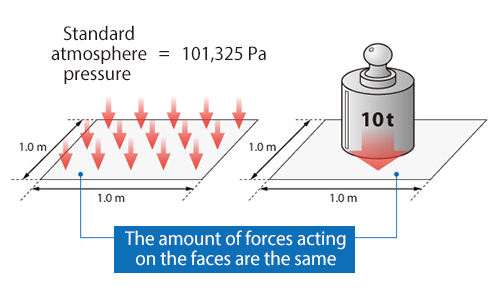# Pressure

## Pressure

Pressure is the force acting per unit area.

It can be expressed with the following equation:where;
: Pressure[Pa]
: Force [N]
: Area of the face where force is imposed [m2]